# 集合的学习

• 数组是容器，同样集合也是容器
• 数组可以指定类型，同样集合也可以指定类型
• 数组长度是固定的不能改变，集合的长度是不定的可以改变

## 集合简介：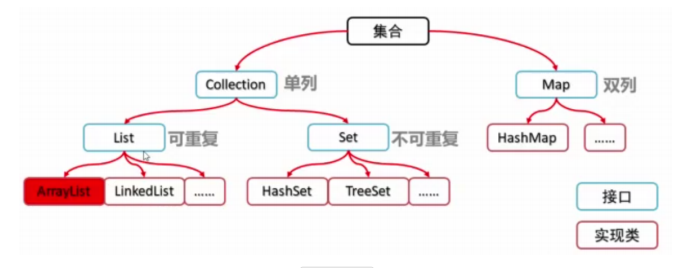## List接口：（单列有序集合）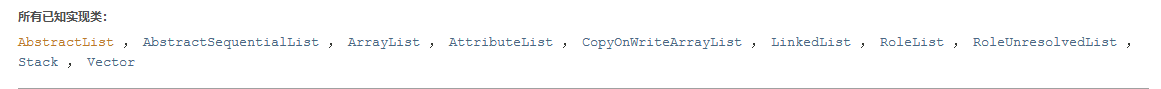### ArrayList实现类

ArrayList的定义（如图所示：ArrayList有三个构造方法）：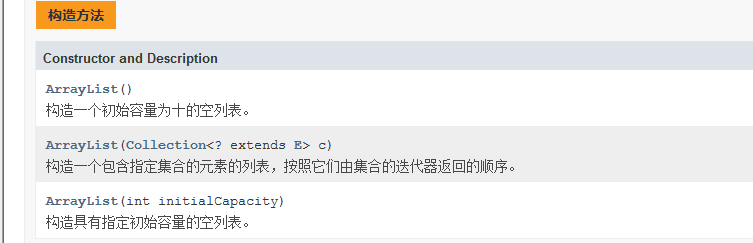public static void main(String[] args) {
/* 第一种： 构造一个初始量为默认的集合 */
ArrayList<String> arrayList = new ArrayList<String>();
/* 第一种： 构造一个添加指定集合的ArrayList集合 */
ArrayList<String> arrayList2 = new ArrayList<String>(arrayList) ;
/* 第三种： 构造一个初始量为20的集合 */
ArrayList<String> arrayList3 = new ArrayList<String>(20);
}

ArrayList使用方法：

• 导包：import java.util.ArrayList;
• 创建对象：ArrayList<String> arrayList = new ArrayList<String>();

ArrayList常用方法：

• clear()：清除集合所有元素。
• contains(Object o):判断集合是否包含该元素，包含返回true，不包含返回false；isEmpty()：判断元素是否不包含，与contains相反
• forEach(Consumer<? super E> action)：对集合遍历操作。
• indexOf(Object o)：返回元素索引。
• iterator()：迭代器遍历。
• remove():删除指定索引位置的元素；remove(Object o)：删除指定元素，如果该元素有多个，则删除第一次出现的那个。
• set(int index, E element)：修改指定索引处的元素。
• size()：返回集合列表长度。

public class ArrayListTest {
public static void main(String[] args) {
ArrayList<Integer> arryList = new ArrayList<Integer>(); // 创建一个默认容量为10的集合,类型为Integer
// 添加方法使用

// 遍历集合方法
arryList.forEach((s) -> System.out.print(s + "  ")); // forEach遍历加lamdba表达式

// 是否包含方法
System.out.println(arryList.contains(21)); // 集合是否包含21，返回false

// set修改方法
arryList.set(0, 7); // 修改索引为0的元素：把67修改为7

// iterator 迭代器遍历
Iterator<Integer> it = arryList.iterator();
System.out.print("迭代器遍历：");
while(it.hasNext()) {
System.out.print(it.next()+"  ");
}
//返回元素12的下标索引
System.out.println("\n12的索引："+arryList.indexOf(12));

//remove元素删除方法
arryList.remove(0);  //根据下标删除 返回布尔类型
arryList.remove("7");  //根据内容删除元素，删除元素为7的元素。返回布尔型

//clear()清除所有元素方法
arryList.clear();

//isEmpty 判空方法
boolean empty = arryList.isEmpty();
if (empty) {
System.out.println("该集合为空");
}
}
}

ArrayList存储类型：

ArrayList对象不能存储基本类型，只能存储引用类型的数据。类似 不能写，但是存储基本数据类型对应的 包装类型是可以的。所以，想要存储基本类型数据， <> 中的数据类型，必须转换后才能编写，转换写法如下：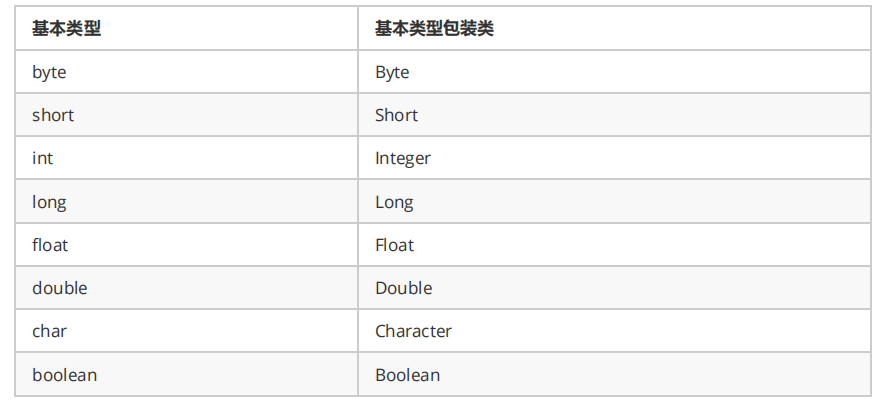ArrayList对象类型操作：

public class ArrayListTest02 {
public static void main(String[] args) {
// 创建存储学生类的集合
ArrayList<Student> studentList = new ArrayList<Student>();
//添加数据
//打印：Student [name=张三, age=21]Student [name=李四, age=12]Student [name=王五, age=34]
studentList.forEach((s) -> System.out.print(s));

//同样一系列的增删查改操作都可以在这里使用
}
}

//实体类 Student
class Student {
private String name;
private int age;

public Student(String name, int age) {
super();
this.name = name;
this.age = age;
}

public Student() {

}

public String getName() {
return name;
}

public void setName(String name) {
this.name = name;
}

public int getAge() {
return age;
}

public void setAge(int age) {
this.age = age;
}

@Override
public String toString() {
return "Student [name=" + name + ", age=" + age + "]";
}

}

• addFirst(E e)：从集合开头插入元素。
• addLast(E e)：将指定元素添加到集合尾部。
• get(int index)：根据索引返回元素；getFirst()：返回列表第一个元素；getLast()：返回列表最后一个元素。
• offer(E e)：将指定元素添加为集合的最后的一个元素；offerFirst(E e)：在此集合头部添加指定元素；offerLast(E e)：在集合尾部添加元素。
• removeFirst()：删除列表第一个元素，并返回该元素；removeLast()：删除列表最后一个元素，并返回该元素。

public class LinkedListTest {
public static void main(String[] args) {
//创建对象
System.out.println("初始化列表");

System.out.println("获取元素");

//删除
System.out.println("删除后还剩余");
}
}

## set接口（单列无序集合）

set和list的区别：最主要的区别就是有序和无序，两个集合在添加数据时，list集合按照添加顺序依次添加，而set集合则会打乱添加顺序。演示如下：

public class HashSetTest {
public static void main(String[] args) {
ArrayList<Number> arrList = new ArrayList<Number>();
System.out.println("list集合打印：");
arrList.forEach((s) -> System.out.println(s + "  "));
/*
* list集合打印： Number [i=1] Number [i=2] Number [i=3] Number [i=4]
*/
HashSet<Number> hashSet = new HashSet<Number>();
System.out.println("set集合打印：");
hashSet.forEach((s) -> System.out.println(s + "  "));
/*
* set集合打印： Number [i=3] Number [i=4] Number [i=1] Number [i=2]
*/

}
}

class Number {
int i;

public Number(int i) {
this.i = i;
}

@Override
public String toString() {
return "Number [i=" + i + "]";
}

}

### HashSet实现类

hashSet集合底层存储结构：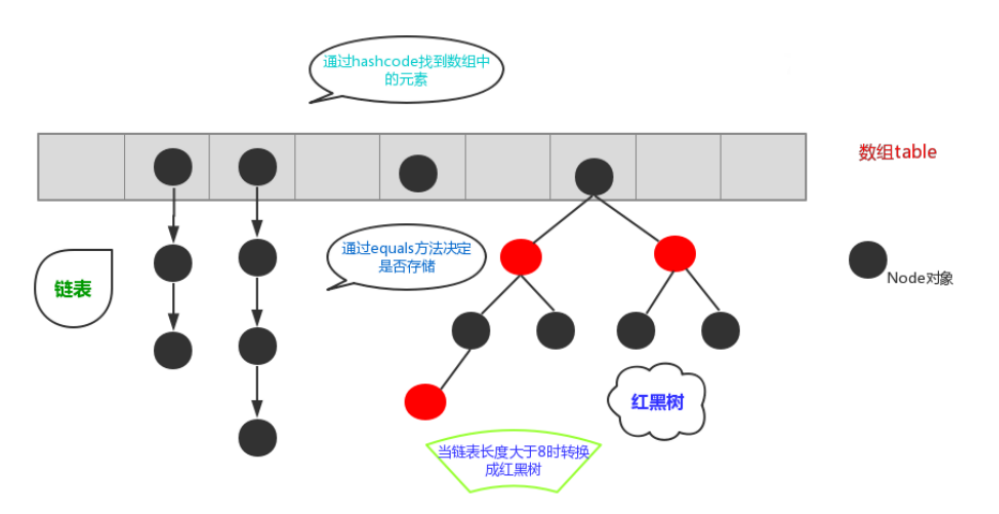equals和hashCode方法的作用

public class HashSetTest {
public static void main(String[] args) {
HashSet<Number> hashSet = new HashSet<Number>();
System.out.println("set集合打印：");
hashSet.forEach((s) -> System.out.println(s + "  "));

/* 重写前：
* set集合打印： Number [i=3] Number [i=4] Number [i=1] Number [i=2]
*/

/*重写后：
* set集合打印： Number [i=1] Number [i=2] Number [i=3] Number [i=4]
*/
}
}

class Number {
int i;

public Number(int i) {
this.i = i;
}

@Override
public String toString() {
return "Number [i=" + i + "]";
}

@Override
public int hashCode() {
final int prime = 31;
int result = 1;
result = prime * result + i;
return result;
}

@Override
public boolean equals(Object obj) {
if (this == obj)
return true;
if (obj == null)
return false;
if (getClass() != obj.getClass())
return false;
Number other = (Number) obj;
if (i != other.i)
return false;
return true;
}

}

equals判断两个对象内容是否相等，hashCode判断两个对象地址值是否相等，这也间接的是set集合不会出现重复值，除去自己定义的引用类外，jdk里面的引用数据类型，也默认重写了这两个方法。（这也是set集合和list集合的一大区别）：

    public static void main(String[] args) {
ArrayList<Integer> arrayList = new ArrayList<Integer>();
arrayList.forEach(System.out::println);//打印了12,2,12允许重复值出现
System.out.println("-------------------------");
HashSet<Integer> hashSet = new HashSet<Integer>();
hashSet.forEach(System.out::println);//打印了2 12，默认去重
}

public static void main(String[] args) {
}

## Map（双列集合）

map引入：现实生活中，我们常会看到这样的一种集合：IP地址与主机名，身份证号与个人，系统用户名与系统用户对象等，这种一一对应的关系，就叫做映射。Java提供了专门的集合类用来存放这种对象关系的对象，即 java.util.Map 接口。

### map常用实现类：

• HashMap<K,V>：存储数据采用的哈希表结构，元素的存取顺序不能保证一致。由于要保证键的唯一、不重复，需要重写键的hashCode()方法、equals()方法。

### HashMap<K,V>实现类

hashMap常用方法：

• public V put(K key, V value) : 把指定的键与指定的值添加到Map集合中。
• public V remove(Object key) : 把指定的键 所对应的键值对元素 在Map集合中删除，返回被删除元素的值。
• public V get(Object key) 根据指定的键，在Map集合中获取对应的值。
• boolean containsKey(Object key) 判断集合中是否包含指定的键。
• public Set<K> keySet() : 获取Map集合中所有的键，存储到Set集合中。
• public Set<Map.Entry<K,V>> entrySet() : 获取到Map集合中所有的键值对对象的集合(Set)
• public Set<K> keySet()：获取map中的所有key值存储到set集合并返回。

hashMap示列代码：

public class HashMapTest {
public static void main(String[] args) {
HashMap<Integer, String> hashMap = new HashMap<Integer, String>(); //定义一个key为Integr类型，values为String类型的HashMap

//添加方法
hashMap.put(1, "张三");
hashMap.put(2, "李四");
hashMap.put(3, "王五");
hashMap.forEach((k,v) ->System.out.println(k+"="+v));

//keySet方法
Set<Integer> keySet = hashMap.keySet();//返回包含集合中所有key值的集合
keySet.forEach(System.out::print);

}
}

hashMap的特点：

• 存在 k v两个参数值
• key值不能重复，如有两个相同，后者会覆盖前者的值
• HashMap由数组+链表组成的
• 存储的值不是有序的
• 在使用自定义的对象当参数时，需要重写equals和hashCode方法

public class LinkedHashMapTest {
public static void main(String[] args) {
HashMap<Integer, String> hashMap = new HashMap<Integer, String>();
hashMap.put(52, "张三");
hashMap.put(9, "李四");
hashMap.put(21, "王五");
hashMap.forEach((k,v) ->{System.out.println(k+"="+v);});
/* 随机存储
* 52=张三 21=王五 9=李四
*/

/*有序存储
* 52=张三 9=李四 21=王五
*/
}
}

### 集合综合案例：

1. 按照斗地主的规则，完成洗牌发牌的动作。
2. 组装54张扑克牌将
3. 54张牌顺序打乱
4. 三个玩家参与游戏，三人交替摸牌，每人17张牌，最后三张留作底牌。
5. 查看三人各自手中的牌（按照牌的大小排序）、底牌

public class Poker {
public static void main(String[] args) { /** 1组装54张扑克牌 */ // 1.1 创建Map集合存储
Map<Integer, String> pokerMap = new HashMap<Integer, String>();
// 1.2 创建 花色集合 与 数字集合
List<String> colors = new ArrayList<String>();
List<String> numbers = new ArrayList<String>();
// 1.3 存储 花色 与数字
Collections.addAll(numbers, "2", "A", "K", "Q", "J", "10", "9", "8", "7", "6", "5", "4", "3"); // 设置 存储编号变量
int count = 1;
pokerMap.put(count++, "大王");
pokerMap.put(count++, "小王"); // 1.4 创建牌 存储到map集合中
for (String number : numbers) {
for (String color : colors) {
String card = color + number;
pokerMap.put(count++, card);/** 2 将54张牌顺序打乱 */ // 取出编号 集合
}
}
Set<Integer> numberSet = pokerMap.keySet(); // 因为要将编号打乱顺序 所以 应该先进行转换到 list集合中
List<Integer> numberList = new ArrayList<Integer>();

// 打乱顺序
Collections.shuffle(numberList); // 3 完成三个玩家交替摸牌，每人17张牌，最后三张留作底牌
// 3.1 发牌的编号
// 创建三个玩家编号集合 和一个 底牌编号集合
List<Integer> noP1 = new ArrayList<Integer>();
List<Integer> noP2 = new ArrayList<Integer>();
List<Integer> noP3 = new ArrayList<Integer>();
List<Integer> dipaiNo = new ArrayList<Integer>(); // 3.2发牌的编号
for (int i = 0; i < numberList.size(); i++) { // 获取该编号
Integer no = numberList.get(i); // 发牌 // 留出底牌
if (i >= 51) {
} else {
if (i % 3 == 0) {
} else if (i % 3 == 1) {
}
}
}// 4 查看三人各自手中的牌（按照牌的大小排序）、底牌

// 4.1 对手中编号进行排序
Collections.sort(noP1);
Collections.sort(noP2);
Collections.sort(noP3);
Collections.sort(dipaiNo); // 4.2 进行牌面的转换
// 创建三个玩家牌面集合 以及底牌牌面集合
List<String> player1 = new ArrayList<String>();
List<String> player2 = new ArrayList<String>();
List<String> player3 = new ArrayList<String>();
List<String> dipai = new ArrayList<String>();
// 4.3转换
for (Integer i : noP1) {
// 4.4 根据编号找到 牌面
pokerMap String card = pokerMap.get(i);
// 添加到对应的 牌面集合中
}
for (Integer i : noP2) {
String card = pokerMap.get(i);
}for (Integer i : noP3) {
String card = pokerMap.get(i);
}
for (Integer i : dipaiNo) {
String card = pokerMap.get(i);
}
//4.5 查看
System.out.println("令狐冲："+player1);
System.out.println("石破天："+player2);
System.out.println("鸠摩智："+player3);
System.out.println("底牌："+dipai); }

}

posted @ 2020-08-13 20:47  一生的风景  阅读(90)  评论(0编辑  收藏